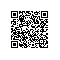# 探索前端黑科技——通过png图的rgba值缓存数据1. const fs = require('fs'
2.
3. const http = require('http'
4.
5. const url = require('url'
6.
7. const querystring = require('querystring'
8.
9. const util = require('util'
10.
11.
12.
13. const server = http.createServer((req, res) => {
14.
15.   let pathname = url.parse(req.url).pathname
16.
17.   let realPath = 'assets' + pathname
18.
19.   console.log(realPath)
20.
21.   if (realPath !== 'assets/upload') {
22.
24.
25.       if (err) {
26.
28.
29.         res.end(err)
30.
31.       } else {
32.
34.
35.           'Access-Control-Allow-Origin''*'
36.
37.           'Content-Type''image/png'
38.
39.           'ETag'"666666"
40.
41.           'Cache-Control''public, max-age=31536000'
42.
43.           'Expires''Mon, 07 Sep 2026 09:32:27 GMT'
44.
45.         })
46.
47.         res.write(file, "binary"
48.
49.         res.end()
50.
51.       }
52.
53.    })
54.
55.   } else {
56.
57.     let post = ''
58.
59.     req.on('data', (chunk) => {
60.
61.       post += chunk
62.
63.     })
64.
65.     req.on('end', () => {
66.
67.       post = querystring.parse(post)
68.
69.       console.log(post.imgData)
70.
72.
73.         'Access-Control-Allow-Origin''*'
74.
75.       })
76.
77.       let base64Data = post.imgData.replace(/^data:image\/\w+;base64,/, ""
78.
79.       let dataBuffer = new Buffer(base64Data, 'base64'
80.
81.       fs.writeFile('assets/out.png', dataBuffer, (err) => {
82.
83.         if (err) {
84.
85.           res.write(err)
86.
87.           res.end()
88.
89.         }
90.
91.         res.write('OK'
92.
93.         res.end()
94.
95.       })
96.
97.     })
98.
99.   }
100.
101. })
102.
103.
104.
105. server.listen(80)
106.
107.
108.
109. console.log('Listening on port: 80')

2.
3.   'Access-Control-Allow-Origin''*'
4.
5.   'Content-Type''image/png'
6.
7.   'ETag'"666666"
8.
9.   'Cache-Control''public, max-age=31536000'
10.
11.   'Expires''Mon, 07 Sep 2026 09:32:27 GMT'
12.
13. })

1. <!-- client.html -->
2.
3.
4.
5. <canvas id="canvas" width="8", height="1"></canvas>

1. <!-- client.js -->
2.
3.
4.
5. let keyString = '01234567890123456789012345678901'
6.
7.
8.
9. let canvas = document.querySelector('#canvas'
10.
11. let ctx = canvas.getContext('2d'
12.
13.
14.
15. let imgData = ctx.createImageData(8, 1)
16.
17.
18.
19. for (let i = 0; i < imgData.data.length; i += 4) {
20.
21.     imgData.data[i + 0] = parseInt(keyString[i]) + 50
22.
23.     imgData.data[i + 1] = parseInt(keyString[i + 1]) + 100
24.
25.     imgData.data[i + 2] = parseInt(keyString[i + 2]) + 150
26.
27.     imgData.data[i + 3] = parseInt(keyString[i + 3]) + 200
28.
29.
30.
31.
32. ctx.putImageData(imgData, 0, 0)

1. // console.log(imgData.data)
2.
3. [50, 101, 152, 203, 54, 105, 156, 207, 58, 109, 150, 201, 52, 103, 154, 205, 56, 107, 158, 209, 50, 101, 152, 203, 54, 105, 156, 207, 58, 109, 150, 201]

1. \$.post('http://xx.xx.xx.xx:80/upload', { imgData: canvas.toDataURL() }, (data) => {
2.
3.     if (data === 'OK') {
4.
5.         let img = new Image()
6.
7.         img.crossOrigin = "anonymous"
8.
9.         img.src = 'http://xx.xx.xx.xx:80/out.png'
10.
11.         img.onload = () => {
12.
13.             console.log('完成图片请求与缓存'
14.
15.             ctx.drawImage(img, 0, 0)
16.
17.             console.log(ctx.getImageData(0, 0, 8, 1).data)
18.
19.         }
20.
21.     }
22.
23. })200 OK，证明是从服务端获取的图片。200 OK (from cache)，证明是从本地缓存读取的图片。使用钉钉扫一扫加入圈子
+ 订阅